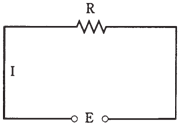## DC circuit Assignment Help

Assignment Help: >> Electrical Engineering - DC circuit

Voltage/current/resistance DC circuit

Most direct current circuits is ultimately boiled down to 3 major components:  a voltage source, a set of conductors, and a resistance. This is shown in schematic diagram of Figure given below. The voltage or EMF source is known as E; the current in conductor is called I; the resistance is called as R. The standard units for these components are voltage, ampere, and ohm respectively.Figure--Simple dc circuit. The voltage is E, the current is I, and the resistance is R.

There prevails a relationship between these 3 quantities. If one of them changes, then one or both of the others will get changed also. If you make the resistance smaller, the current will become larger and if you make the EMF source smaller, the current will decrease automatically. If the current in the circuit increases, voltage across the resistor will also increase. There exists a basic arithmetic relationship between these 3 quantities.